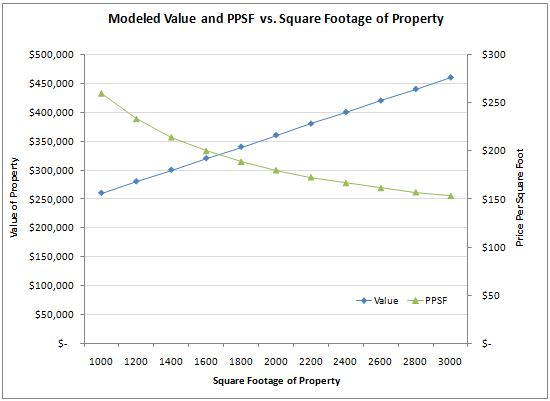What is a hedonic price index?

A hedonic price index is a fundamentally different method for calculating home price trends. The term "hedonic" refers to the concept that the value of a home can be determined by looking at the value of the constituent components of a home. A home can be viewed, mathematically speaking, as a collection of parameters such as (beds=3, baths=2, garages=2, sqft=1800, lotsize=2500, neighborhood=Woodbridge, Irvine, CA, and so forth). With enough data points, over enough time, a regression model can be used to determine the relationship between each of these parameters and the value of a home.

Because a hedonic approach is based on a holistic view of all the data available, it is not inherently skewed by the mix shifting to larger (or smaller) properties. A simple example will illustrate why this is the case. Consider town with only two types of properties: small (1600 sqft) homes and larger (2000 sqft) homes. Small homes have constant value at \$320,000 and large homes have constant value at \$360,000.

Month Sales Mix Median PPSF
May 2011 60% small / 40% large \$320,000 \$200
June 2011 40% small / 60% large \$360,000 \$180

Using the above data, we will find that the median home value increases month-over-month and the median price per square foot decreases month-over-month. In reality, the underlying value of any given home has remained unchanged.

A hedonic approach would run a regression model to fit a relationship between the sales price of a home, the sqft of the home, other factors in the model (omitted for this example), and the time period of the homeís sale. Using notation from the excellent open-source statistics package R, our model would be represented as follows:

fit <- lm ( saleprice ~ sqft + month )

Though foreign looking at first glance, the above line invoked the lm() or linear model function in R to create a linear regression. The goal of the linear regression algorithm is to find the best linear relationship between the sales price of a home, the square footage of the home, and the month of sale. By feeding in many months of data, and asking the regression to compute the impact of each month, we can generate a price trend series (using the regression coefficients for each month). As a technical aside, "month" must be used as a dummy variable, so the actual regression will have one variable for each of the months under analysis (excluding the first month, again for technical reasons).

In the above example, R will report back the impact of square footage and the impact of being in the second month as regression coefficients.

Coefficients:
Estimate  Std. Error t value Pr(>|t|)
(Intercept)     1.600e+05 5.494e-11 2.912e+15 2e-16 ***
qt\$sqft         1.000e+02 3.034e-14 3.296e+15 2e-16 ***
qt\$monthJun2011 NA NA NA NA

R reports a few facts from the above output. First, the month (May vs. June) has no impact. In our situation, this means that prices have not changed from month to month. Second, each square foot of home adds \$100 in value. Third, homes have an "intercept" of \$160,000. Even if a home has zero square footage of size, it's still theoretically worth \$160,000. In practice, such relationships tend to break down (i.e. a linear model is not a correct approximation at the endpoints), but we can ignore that fact for the time being.

Because the regression analysis develops a relationship between the salesprice, the square footage, and the time of sale, trends over time are less subject to distortion. Even the most basic linear regression model is less distorted than both a simple median and a price-per-square-foot (PPSF) approach. The price = c1 + sqft * c2 structure of the equation creates a situation where PPSF naturally declines as size increases. As a simple illustration of this, consider the following chart, based on the above example.What are the weaknesses of hedonic indexes?

We think hedonic real estate indexes are a solid way to measure price movements over time. With that said, they are not perfect (no method is). Hedonic indexes suffer from a few common issues. First, they require a considerable amount of data for each time period, neighborhood, or other factor under consideration. Second, they can't handle data points where even one element is missing or dirty. For example, if the a home sale record lists 3 beds, 2 baths, a 2 car garage, and a total size of 0 sq ft, that record is erroneous that can not be used in the regression model.

Finally, one subtle issue with hedonic regression models is that they assume that the way a market values the components of a property does not change over time. The specific valuation of an element (such as a bathroom) should add about the same \$\$ or % to the worth of a property over time. Over the short-term, consumer preferences and market valuation do not change much. However, for long-term trending, a significant shift in how consumers value a property will cause a distortion in the model. Global Decision is currently analyzing several datasets to determine what, if any, impact preference shifts might produce over the long-term.

Next, tutorial: how to construct a hedonic real estate price index